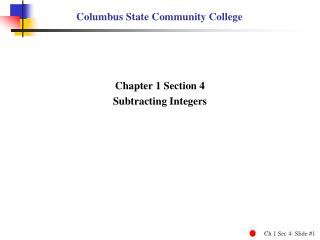DownloadDownload PresentationColumbus State Community College

# Columbus State Community College

Télécharger la présentation## Columbus State Community College

- - - - - - - - - - - - - - - - - - - - - - - - - - - E N D - - - - - - - - - - - - - - - - - - - - - - - - - - -
##### Presentation Transcript

1. Columbus State Community College Chapter 1 Section 4 Subtracting Integers

2. Subtracting Integers • Find the opposite of a signed number. • Subtract integers. • Combine adding and subtracting of integers.

3. Finding the Opposites of Signed Numbers EXAMPLE 1 Finding the Opposites of Signed Numbers Find the opposite (additive inverse) of each number. Show that the sum of the number and its opposite is 0. (a) 9 The opposite of 9 is –9 and 9 + –9 = 0 (b)–14 The opposite of –14 is 14 and –14 + 14 = 0 (c) 0 The opposite of 0 is 0 and 0 + 0 = 0

4. Subtracting Two Integers Subtracting Two Integers To subtract two numbers, add the first number to the opposite of the second number. Remember to change two things: Step 1 Make a pencil stroke to change the subtraction symbol to an addition symbol. Step 2 Make a second pencil stroke to change the second number to its opposite. If the second number is positive, change it to negative. If the second number is negative, change it to positive.

5. CAUTION CAUTION When changing a subtraction problem to an addition problem, do not make any change in the first number. The pattern is 1st number – 2nd number = 1st number + opposite of 2nd number.

6. Subtracting Two Integers EXAMPLE 2 Subtracting Two Integers Make two pencil strokes to change each subtraction problem into an addition problem. Then find the sum. (a) 9 – 15 – – = –6 9 15 + Change 15 to –15 Change subtraction to addition.

7. Subtracting Two Integers EXAMPLE 2 Subtracting Two Integers Make two pencil strokes to change each subtraction problem into an addition problem. Then find the sum. (b)–17 – –8 + = –9 –17 –8 – + Change –8 to +8 Change subtraction to addition.

8. Subtracting Two Integers EXAMPLE 2 Subtracting Two Integers Make two pencil strokes to change each subtraction problem into an addition problem. Then find the sum. 4 – –12 (c) 4 – –12 Change subtraction to addition. 4 + –12 Change –12 to 12. 4 + +12 16

9. Subtracting Two Integers EXAMPLE 2 Subtracting Two Integers Make two pencil strokes to change each subtraction problem into an addition problem. Then find the sum. –3 – 6 (d)–3 – 6 Change subtraction to addition. –3 + 6 Change 6 to –6. –3 + –6 –9

10. Combining Addition and Subtraction EXAMPLE 3 Combining Addition and Subtraction Simplify by completing all the operations. –5 – –10 + –7 – 2 –5 – –10 + –7 – 2 –5 + +10 + –7 + –2 –5 + –10 + –7 + 2 Change –10 to 10. Change 2 to –2. Change subtraction to addition. 5 + –7 + –2 –2 + –2 –4

11. Calculator Tip You can use the negative sign keyon your TI-30X IIScalculator to enter negative numbers. Example 3: –5 – –10 + –7 – 2 (–) – + – 5 10 7 2 = (–) (–) (–) (–) Calculator Tip – TI-30X IIS –4

12. Subtracting Integers Chapter 1 Section 4 – End Written by John T. Wallace